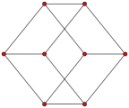## Finding hyperrectangle vertex coordinatesTiny post Python NumPy N-dimensional

For the past two years I have been working on a python library for tiling and merging of n-dimensional numpy arrays, tiler. From the first release it had a method to return bounding boxes of resulting tiles but it returned only minimum (i.e., bottom-left) and maximum (i.e., top-right) corners. Recently I needed to find all vertices of such bounding box, based on two original opposite vertices returned by `Tiler.get_tile_bbox()`.

The solutions that come to mind first are to calculate coordinates with multiple nested for loops. While this part is not very performance critical, I was still interested in trying to rewrite that in numpy to avoid for loops. I found an algorithm on stackoverflow that involved iterating through 2^n numbers and then iterating through each bit of that number in binary. Pretty cool approach, but implementing this straight in Python seemed a nuissance: converting an integer to binary produces a string (i.e., `bin(9) => '0b1001'`), then iterating through that string and checking with and if-else whether it is 0 or 1 and then sampling correct coordinate...

I didn't see any other implementation of this on the internet, so here I am, sharing my Sunday night solution, with just one list comprehension. Essentially it creates an array of combinations of bits up to 2^n and then use it for indexing from interval array.

Probably it can be optimized even further 🤔

``````def get_all_corners(bottom_left_corner, top_right_corner):
n_dim = len(bottom_left_corner)
mins = np.minimum(bottom_left_corner, top_right_corner)
maxs = np.maximum(bottom_left_corner, top_right_corner)
intervals = np.stack([mins, maxs], -1)
indexing_bits = np.array(list(itertools.product([0, 1], repeat=n_dim)))
corners = np.stack([intervals[x][indexing_bits.T[x]] for x in range(n_dim)], -1)
return corners
``````

-- Ilja